# Daniell integral

(diff) ← Older revision | Latest revision (diff) | Newer revision → (diff)

An extension of the concept of the integral, proposed by P. Daniell . The construction scheme of this integral, which is known as the Daniell scheme, is an extension of the integral originally defined for a certain set of functions — the so-called elementary functions — to a wider class of functions. While retaining the way of extending but by changing the content of the initial set of elementary functions, it is possible to arrive at different extensions of the concept of the integral. In this scheme the concept of an elementary integral is axiomatically defined, unlike in Lebesgue's scheme (cf. Lebesgue integral) in which the concept of a measure is axiomatic.

Letbe an arbitrary set and let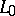be a certain set of real bounded functions defined on; these functions are called elementary. It is assumed thatis a vector lattice, i.e. fromand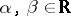it follows that, andLetbe a real functional defined onsuch that

1)(linearity);

2)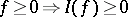(non-negativity);

3) if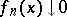for all, then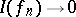(continuity with respect to monotone convergence).

Such a functional is known as an integral over elementary functions or an elementary integral. A setis said to be a set of measure zero if for eachthere exists a non-decreasing sequence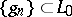such that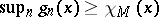for all, andHere,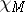denotes the characteristic function of.

A functiondefined onbelongs to the class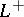if there exists a sequence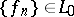such that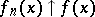almost-everywhere and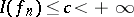. The numberis said to be the integral of. The integraldoes not depend on the choice of the particular approximating sequence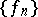.

The classis the set of functionswhich are defined onand which are representable in the form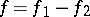, where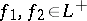. Functions of the classare called summable, while the number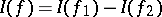is known as the Daniell integral of the function. The classis a vector lattice of finite functions (considered up to sets of measure zero) which is closed with respect to almost-everywhere convergence, with finite integrals, while the Daniell integral of summable functions has the properties of linearity, non-negativity, continuity with respect to almost-everywhere convergence of majorable summable functions (Lebesgue's theorem on passing to the limit under the integral sign), and also several other natural properties of the integral.

If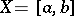andis the set of the step functions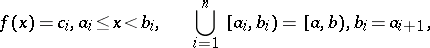the Daniell integral becomes identical with the Lebesgue integral on the summable functions on. The Daniell scheme may be used to construct the integral of functions with values in a-complete lattice.

How to Cite This Entry:
Daniell integral. Encyclopedia of Mathematics. URL: http://encyclopediaofmath.org/index.php?title=Daniell_integral&oldid=17172
This article was adapted from an original article by V.I. Sobolev (originator), which appeared in Encyclopedia of Mathematics - ISBN 1402006098. See original article